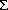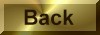Practice Problem 5

CalculateHḞ andSḞ for the following reaction and decide in which direction each of these factors will drive the reaction.

N2(g) + 3 H2(g)2 NH3(g)

Solution

Using a standard-state enthalpy of formation and absolute entropy data table, we find the following information:

CompoundHfo(kJ/mol)         SḞ(J/mol-K)

N2(g)                                 0                 191.61

H2(g)                                 0                 130.68

NH3(g)                             -46.11         192.45

The reaction is exothermic (HḞ < 0), which means that the enthalpy of reaction favors the products of the reaction:Ho =Hfo(products) -Hfo(reactants)

= [2 mol NH3 x 46.11 kJ/mol] - [1 mol N2 x 0 kJ/mol + 3 mol H2 x 0 kJ/mol]

= -92.22 kJ

The entropy of reaction is unfavorable, however, because there is a significant increase in the order of the system,  when  N2 and H2 combine to form NH3.So =So(products) -So(reactants)

= [2 mol NH3 x 192.45 J/mol-K] - [1 mol N2 x 191.61 J/mol-K + 3 mol H2 x 130.68 J/mol-K]

= -198.75 J/K